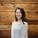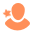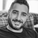## Multilevel dynamic pages with HubDB - Recent products

SOLVEContributor

Hi,

I'm reading through this tutorial, and for the most part, it does what I need it to do

How to build multilevel dynamic pages with HubDB (hubspot.com)

An area, I'm not sure on is recent additions to the DB. What I'm looking to do is create a portion of the page that displays the last 5 additions to any of the HubDB's.

Using that tutorial, you can just loop through all of the Childs DB's and have the page populate with all the products. It's then fairly straight forward to restrict it to only display 5/6 entries. What I'm not sure on is how to only display the last 5 products added to any of the DB's. If this was using a single DB, that's fairly straight forward but I'm not sure how you would accomplish this across multiple Child DB's.

Is that even possible?

Phil

1 Accepted solution

Accepted SolutionsSolution

Yep, exactly 👍

{% do childCombineArr.append({'date': date, 'row': row}) %} is the line that adds the child db row info to the array. 'row' is just all the info in each row, and will be an object itself, but the info you want to sort (in my case date) by has to be added outside of the row object, as it were, to be used by the sort function on childCombineArr. So it returns

``[{date=1594717200000, row={id=[id], createdAt=1597401699900, path='[path]', etc... }]``

For you because you want to sort by the latest 5 products added you could use createdAt instead of date and then use if loop.index is less than or equal to 5 e.g.

``````{% set childCombineArr = [] %}
{% for row in table %}
{% if row.hs_child_table_id %}
{% set child_table = hubdb_table_rows(row.hs_child_table_id) %}
{% for row in child_table %}
{% set timeAdded = row.createdAt %}
{% endfor %}
{% endif %}
{% endfor %}
{% for item in childCombineArr|sort(True, False, 'timeAdded') %}
{% if loop.index <= 5 %}
{% set row = item.row %}
{{row}}
{% endif %}
{% endfor %}``````

7 Replies 7Community Manager

@tjoyce and @thesnappingdog , are you guys familiar with this topic? Would you mind sharing your advice for @Philip_Marsh ?Recognized Expert | Platinum Partner

@piersg - might have some insight on this topicHi @Philip_Marsh (thanks @tjoyce). Something I did to sort multiple child DBs by date could be useful for you. Basically combine them into a single array then sort that resulting array.

``````{% set childCombineArr = [] %}
{% for row in table %}
{% if row.hs_child_table_id %}
{% set child_table = hubdb_table_rows(row.hs_child_table_id) %}
{% for row in child_table %}
{% set date = row.start_datetime %}
{% do childCombineArr.append({'date': date, 'row': row}) %}
{% endfor %}
{% endif %}
{% endfor %}
{% for item in childCombineArr|sort(True, False, 'date') %}
{% set row = item.row %} // allows you to use standard row.title as if it were for "row in table" instead of item.row.title
{{row.title}}
{{row.start_datetime|datetimeformat('%e %B %Y')}}
//...etc
{% endfor %}``````Contributor

Thank you, I'll give that a try.

I haven't array's before now in Hubl. Am I right in breaking this down.

``{% set childCombineArr = [] %}``

This creates an empty Array, we then initiate a loop to go through the child DB's and adds that information to an array.

``{% for item in childCombineArr|sort(True, False, 'date') %}``

We then create a second loop which uses the data in the array to print to the screen, but after it has sorted it by date?

ThanksSolution

Yep, exactly 👍

{% do childCombineArr.append({'date': date, 'row': row}) %} is the line that adds the child db row info to the array. 'row' is just all the info in each row, and will be an object itself, but the info you want to sort (in my case date) by has to be added outside of the row object, as it were, to be used by the sort function on childCombineArr. So it returns

``[{date=1594717200000, row={id=[id], createdAt=1597401699900, path='[path]', etc... }]``

For you because you want to sort by the latest 5 products added you could use createdAt instead of date and then use if loop.index is less than or equal to 5 e.g.

``````{% set childCombineArr = [] %}
{% for row in table %}
{% if row.hs_child_table_id %}
{% set child_table = hubdb_table_rows(row.hs_child_table_id) %}
{% for row in child_table %}
{% set timeAdded = row.createdAt %}
{% endfor %}
{% endif %}
{% endfor %}
{% for item in childCombineArr|sort(True, False, 'timeAdded') %}
{% if loop.index <= 5 %}
{% set row = item.row %}
{{row}}
{% endif %}
{% endfor %}``````Contributor

Thank you. PerfectCommunity Manager

Thank you so much @tjoyce and @piersg !!